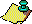Contents Next: Modular Equations and Up: RamanujanModular Equations, Previous: The Miracle of

# Ramanujan's Solvable Modular Equations

Hardy  commenting on Ramanujan's work on elliptic and modular functions says
It is here that both the profundity and limitations of Ramanujan's knowledge stand out most sharply.
We present only one of Ramanujan's modular equations.

### Theorem 2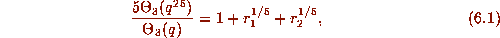where for i=1 and 2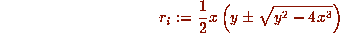with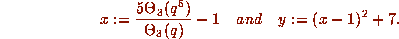This slightly rewritten form of entry 12(iii) of Chapter 19 of Ramanujan's Second Notebook (see , where Berndt's proofs may be studied). One can think of Ramanujan's quintic modular equation as an equation in the multiplier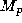of (5.13). The initial surprise is that it is solvable. The quintic modular relation for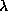,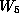, and the related equation for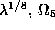, are both nonsolvable. The Galois group of the sixth-degree equation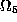(see (5.12)) over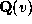is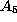and is nonsolvable. Indeed both Hermite and Kronecker showed, in the middle of the last century, that the solution of a general quintic may be effected in terms of the solution of the 5th-order modular equation (5.12) and the roots may thus be given in terms of the theta functions.

In fact, in general, the Galois group for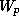of (5.11) has order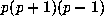and is never solvable for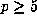. The group is quite easy to compute, it is generated by two permutations. If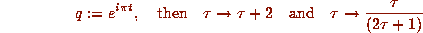are both elements of the-group and induce permutations on the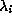of Theorem 1. For any fixed p, one can use the q-expansion of (5.10) to compute the effect of these transformations on the, and can thus easily write down the Galois group. While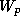is not solvable over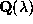, it is solvable over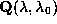. Note that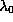is a root of. It is of degree p+1 becauseis irreducible. Thus the Galois group forover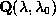has order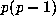. For p=5,7, and 11 this gives groups of order 20, 42, and 110, respectively, which are obviously solvable and, in fact, for general primes, the construction always produces a solvable group.

From (5.8) and (5.10) one sees that Ramanujan's modular equation can be rewritten to give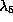solvable in terms ofand. Thus, we can hope to find an explicit solvable relation for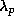in terms ofand. For p=3,is of degree 4 and is, of course, solvable. For p=7, Ramanujan again helps us out, by providing a solvable seventh-order modular identity for the closely related eta function defined by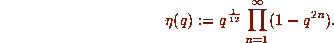The first interesting prime for which an explicit solvable form is not known is the ``endecadic''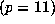case. We consider only prime values because for nonprime values the modular equation factors.

This leads to the interesting problem of mechanically constructing these equations. In principle, and to some extent in practice, this is a purely computational problem. Modular equations can be computed fairly easily from (5.11) and even more easily in the associated variables u and v. Because one knows a priori bounds on the size of the (integer) coefficients of the equations one can perform these calculations exactly. The coefficients of the equation, in the variables u and v, grow at most like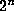. (See .) Computing the solvable forms and the associated computational problems are a little more intricate --- though still in principle entirely mechanical. A word of caution however: in the variables u and v the endecadic modular equation has largest coefficient 165, a three digit integer. The endecadic modular equation for the intimately related function J {Klein's absolute invariant) has coefficients as large as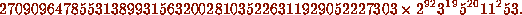It is, therefore, one thing to solve these equations, it is entirely another matter to present them with the economy of Ramanujan.

The paucity of Ramanujan's background in complex analysis and group theory leaves open to speculation Ramanujan's methods. The proofs given by Berndt are difficult. In the seventh-order case, Berndt was aided by MACSYMA --- a sophisticated algebraic manipulation package. Berndt comments after giving the proof of various seventh-order modular identities:

Of course, the proof that we have given is quite unsatisfactory because it is a verification that could not have been achieved without knowledge of the result. Ramanujan obviously possessed a more natural, transparent, and ingenious proof.Contents Next: Modular Equations and Up: RamanujanModular Equations, Previous: The Miracle of